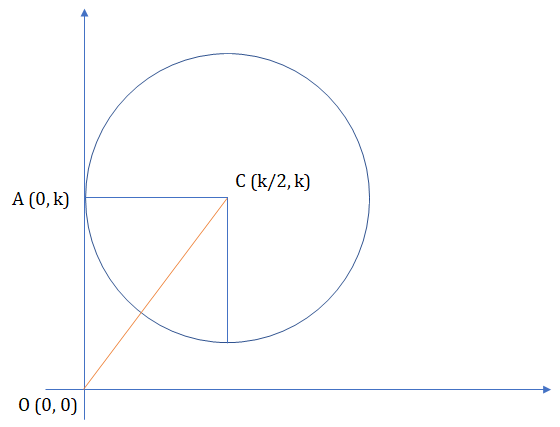Summer is Coming! Join the Game of Timers Competition to Win Epic Prizes. Registration is Open. Game starts Mon July 1st.

 It is currently 20 Jul 2019, 16:01### GMAT Club Daily Prep

#### Thank you for using the timer - this advanced tool can estimate your performance and suggest more practice questions. We have subscribed you to Daily Prep Questions via email.

Customized
for You

we will pick new questions that match your level based on your Timer History

Track

every week, we’ll send you an estimated GMAT score based on your performance

Practice
Pays

we will pick new questions that match your level based on your Timer History

#### Not interested in getting valuable practice questions and articles delivered to your email? No problem, unsubscribe here.# A circle with center (h, k), in which k = 2h, lies tangent to the y-ax

Author Message
TAGS:

### Hide Tags

Senior RC ModeratorV
Status: ----__----___-----____-----
Joined: 02 Nov 2016
Posts: 3071
Location: Pakistan
GPA: 3.39
A circle with center (h, k), in which k = 2h, lies tangent to the y-ax  [#permalink]

### Show Tags

200:00

Difficulty:25% (medium)

Question Stats:76% (01:59) correct24% (02:11) wrongbased on 80 sessions

### HideShow timer StatisticsA circle with center (h, k), in which k = 2h, lies tangent to the y-axis in in the (x, y) coordinate system. What is the distance from the origin to the center of the circle, in terms of k ?

A. $$(k\sqrt{3)}/2$$
B. $$(k\sqrt{5)}/2$$
C. k
D. k $$\sqrt{3}$$
E. k $$\sqrt{5}$$

_________________
New Project RC Butler 2019 - Practice 2 RC Passages Everyday
Final days of the GMAT Exam? => All GMAT Flashcards.
This Post Helps = Press +1 Kudos
Best of Luck on the GMAT!!
Math ExpertV
Joined: 02 Aug 2009
Posts: 7764
Re: A circle with center (h, k), in which k = 2h, lies tangent to the y-ax  [#permalink]

### Show Tags

1
A circle with center (h, k), in which k = 2h, lies tangent to the y-axis in in the (x, y) coordinate system. What is the distance from the origin to the center of the circle, in terms of k ?

A. $$(k\sqrt{3)}/2$$
B. $$(k\sqrt{5)}/2$$
C. k
D. k $$\sqrt{3}$$
E. k $$\sqrt{5}$$

Let us draw the sketch first as per the info..

If we can draw the sketch, we are almost 90% there..Tangent means that a point of the circle lies on the y-axis.
This point on y-axis, the center of the circle and the origin will make a RIGHT angled triangle.

Distance = $$\sqrt{k^2+h^2}=\sqrt{k^2+(k/2)^2}=\sqrt{5k^2/4}=\sqrt{5}*\frac{k}{2}$$

B
Attachments00000.png [ 28.21 KiB | Viewed 787 times ]

_________________
e-GMAT RepresentativeV
Joined: 04 Jan 2015
Posts: 2943
A circle with center (h, k), in which k = 2h, lies tangent to the y-ax  [#permalink]

### Show Tags

1

Solution

Given
• A circle is present in the (x, y) coordinate system, whose center is (h, k)
• The circle is tangent to the y-axis
• Also, h = 2k, or k = h/2

To Find
• The distance from the origin to the center of the circle, in terms of k

Approach & WorkingAs we can see in the figure, angle OAC = 90° (as the circle is tangent to the y-axis)

Therefore, as per Pythagoras Theorem,
• $$OC^2 = OA^2 + AC^2 = k^2 + \frac{k^2}{4} = \frac{5k^2}{4}$$
Or, OC = k$$\sqrt{5}/2$$

Hence, the correct answer is option B.

_________________

Originally posted by EgmatQuantExpert on 10 Feb 2019, 04:09.
Last edited by EgmatQuantExpert on 13 Feb 2019, 22:24, edited 1 time in total.
GMAT Club LegendD
Joined: 18 Aug 2017
Posts: 4252
Location: India
Concentration: Sustainability, Marketing
GPA: 4
WE: Marketing (Energy and Utilities)
Re: A circle with center (h, k), in which k = 2h, lies tangent to the y-ax  [#permalink]

### Show Tags

1
A circle with center (h, k), in which k = 2h, lies tangent to the y-axis in in the (x, y) coordinate system. What is the distance from the origin to the center of the circle, in terms of k ?

A. $$(k\sqrt{3)}/2$$
B. $$(k\sqrt{5)}/2$$
C. k
D. k $$\sqrt{3}$$
E. k $$\sqrt{5}$$

k=2h
center ( h,k)
use the distance formula Sqrt( y2-y1)^2+(x2-x1)^2
and determine the distance from origin (0,0) to center of circle
= > k * sqrt5/2
IMO B
_________________
If you liked my solution then please give Kudos. Kudos encourage active discussions.
Senior ManagerP
Status: Gathering chakra
Joined: 05 Feb 2018
Posts: 369
Re: A circle with center (h, k), in which k = 2h, lies tangent to the y-ax  [#permalink]

### Show Tags

1) Draw a diagram to get an idea of how to solve
2) Plug in numbers for h and k, h=2 k=4
3) Solve using pythagorean theorem, 2²+4² = hypotenuse² --> hyp = 2√5
4) Plug into answers, if k=4 then it's clearly B.Re: A circle with center (h, k), in which k = 2h, lies tangent to the y-ax   [#permalink] 21 Mar 2019, 05:43
Display posts from previous: Sort by

# A circle with center (h, k), in which k = 2h, lies tangent to the y-ax